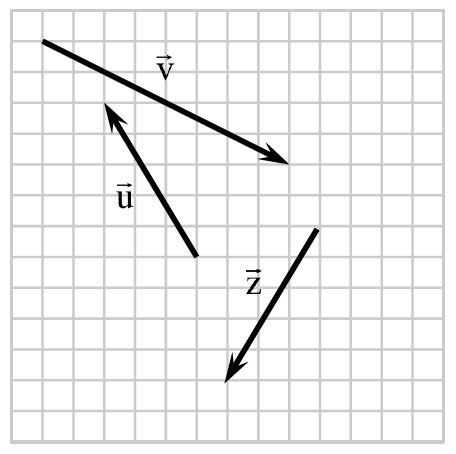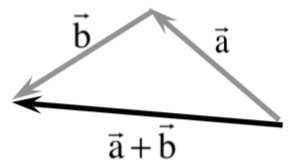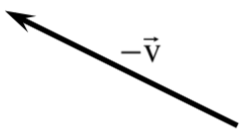### Home > PC3 > Chapter 6 > Lesson 6.2.2 > Problem6-87

6-87.On graph paper, copy the vectors shown at right. Draw and label each of the following vectors. Leave room to separate each answer from the others.

1. $\vec { \text{p} }$, a vector equivalent to $\vec { \text{v} }$.

Vector $\text{p}$ will be the same as vector $\text{v}$, it just needs to begin in a different location.

2. $\vec { \text{u} }+\vec{\text{z}}$3. $\frac{1}{2}\vec{\text{v}}$
This vector will have the same direction as vector $\text{v}$, but it will be half as long.
4. $\vec{\text{u}}-\vec{\text{v}}$
$\text{u − v = u + (−v)}$